# How to Calculate and Solve for Percentage Yield, Actual Yield and Theoretical Yield in Chemistry | The Calculator Encyclopedia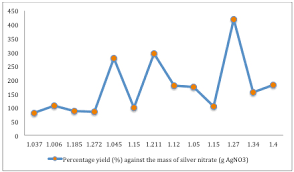The image above represents percentage yield.

To compute the percentage yield, two essential parameters are needed and these parameters are actual yield and theoretical yield.

The formula for calculating percentage yield:

%Yield = (Actual YieldTheoretical Yield) x 100

Let’s solve an example;
Find the percentage yield when the actual yield is 12 with a theoretical yield of 26.

This implies that;
Actual yield = 12
Theoretical yield = 26

%Yield = (Actual YieldTheoretical Yield) x 100
%Yield = (1226) x 100
%Yield = 0.46 x 100
%Yield = 46.15

Therefore, the percentage yield is 46.15.

Calculating the Actual Yield when the Percentage Yield and the Theoretical Yield is Given.

Actual yield = %yield x theoretical yield / 100

Let’s solve an example;
Find the actual yield when the %yield is 52 with a theoretical yield of 10.

This implies that;
%yield = 52
Theoretical yield = 10

Actual yield = %yield x theoretical yield / 100
Actual yield = 52 x 10 / 100
Actual yield = 520 / 100
Actual yield = 5.2

Therefore, the actual yield is 5.2.

Calculating the Theoretical Yield when the Percentage Yield and the Actual Yield is Given.

Theoretical yield = %yield x actual yield / 100

Let’s solve an example;
Find the theoretical yield when the %yield is 60 with a actual yield of 8.

This implies that;
%yield = 60
Actual yield = 8

Theoretical yield = %yield x actual yield / 100
Theoretical yield = 60 x 8 / 100
Theoretical yield = 480 / 100
Theoretical yield = 4.8

Therefore, the theoretical yield is 4.8.

Nickzom Calculator – The Calculator Encyclopedia is capable of calculating the percentage yield.

To get the answer and workings of the percentage yield using the Nickzom Calculator – The Calculator Encyclopedia. First, you need to obtain the app.

You can get this app via any of these means:

To get access to the professional version via web, you need to register and subscribe for NGN 1,500 per annum to have utter access to all functionalities.
You can also try the demo version via https://www.nickzom.org/calculator

Once, you have obtained the calculator encyclopedia app, proceed to the Calculator Map, then click on Basic Chemistry under Chemistry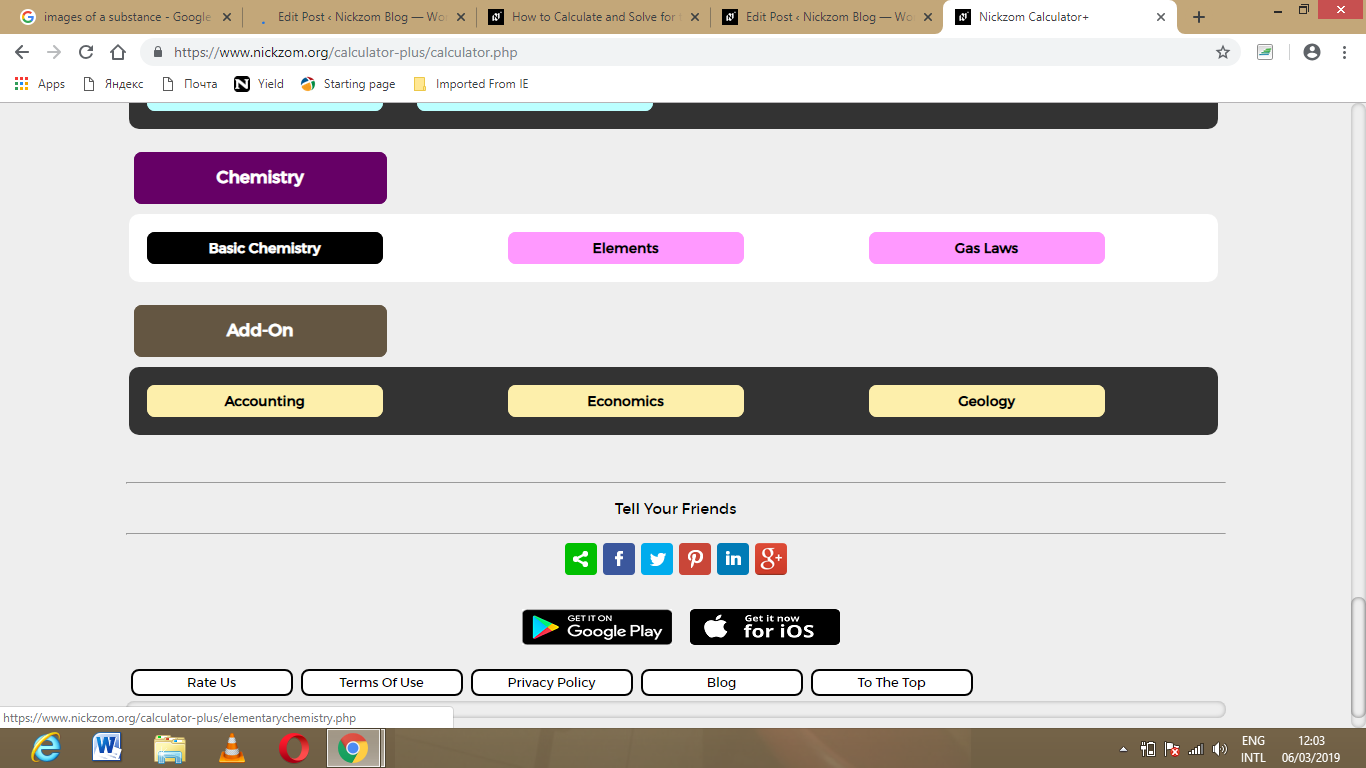Now, Click on Yield under Basic Chemistry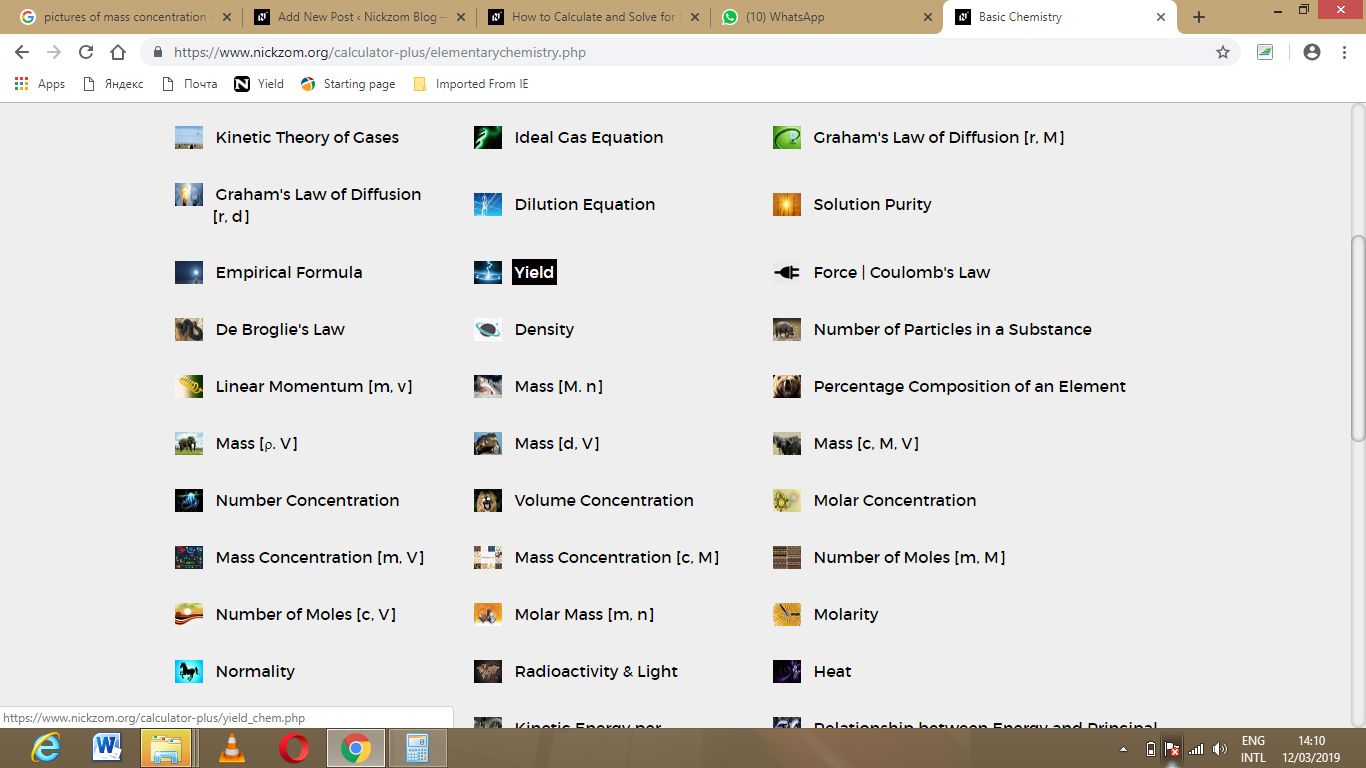Click on Percentage Yield under Yield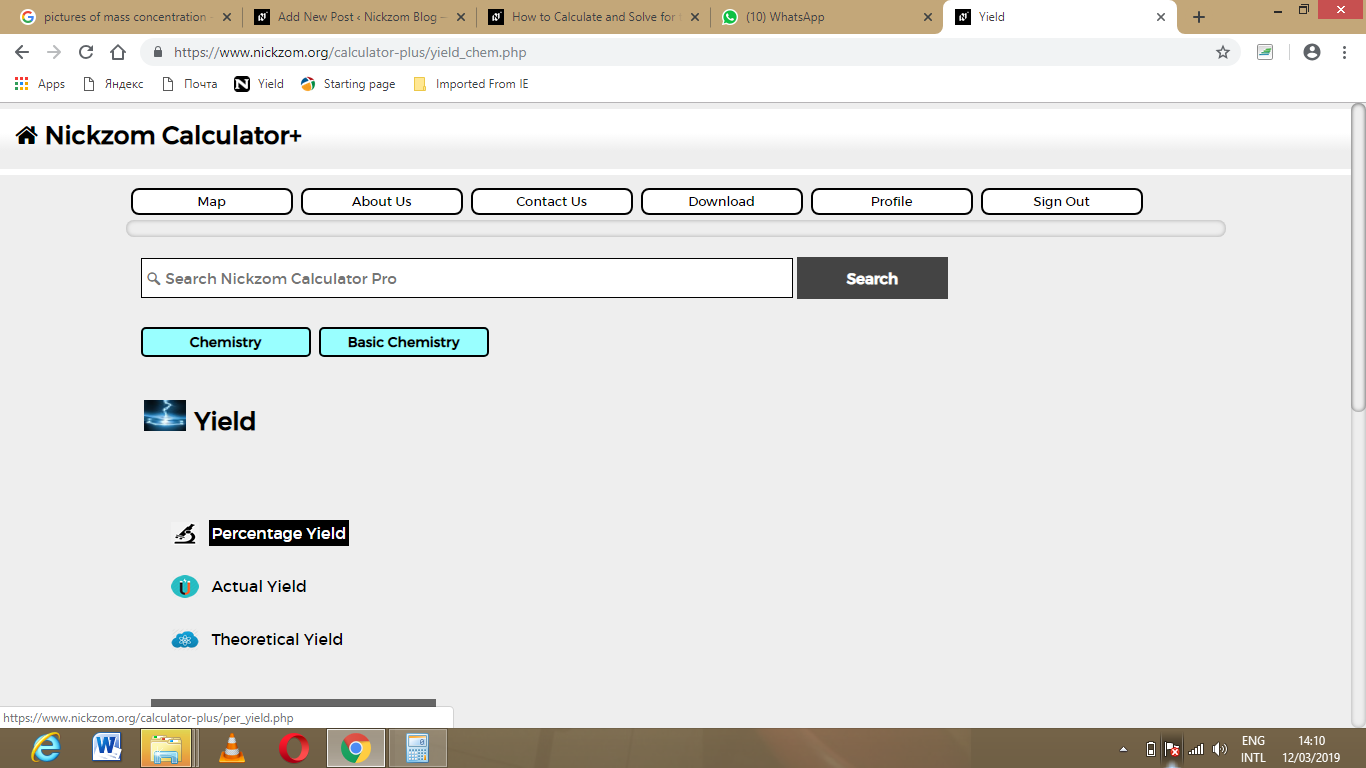The screenshot below displays the page or activity to enter your values, to get the answer for the percentage yield according to the respective parameters which are the actual yield and theoretical yield.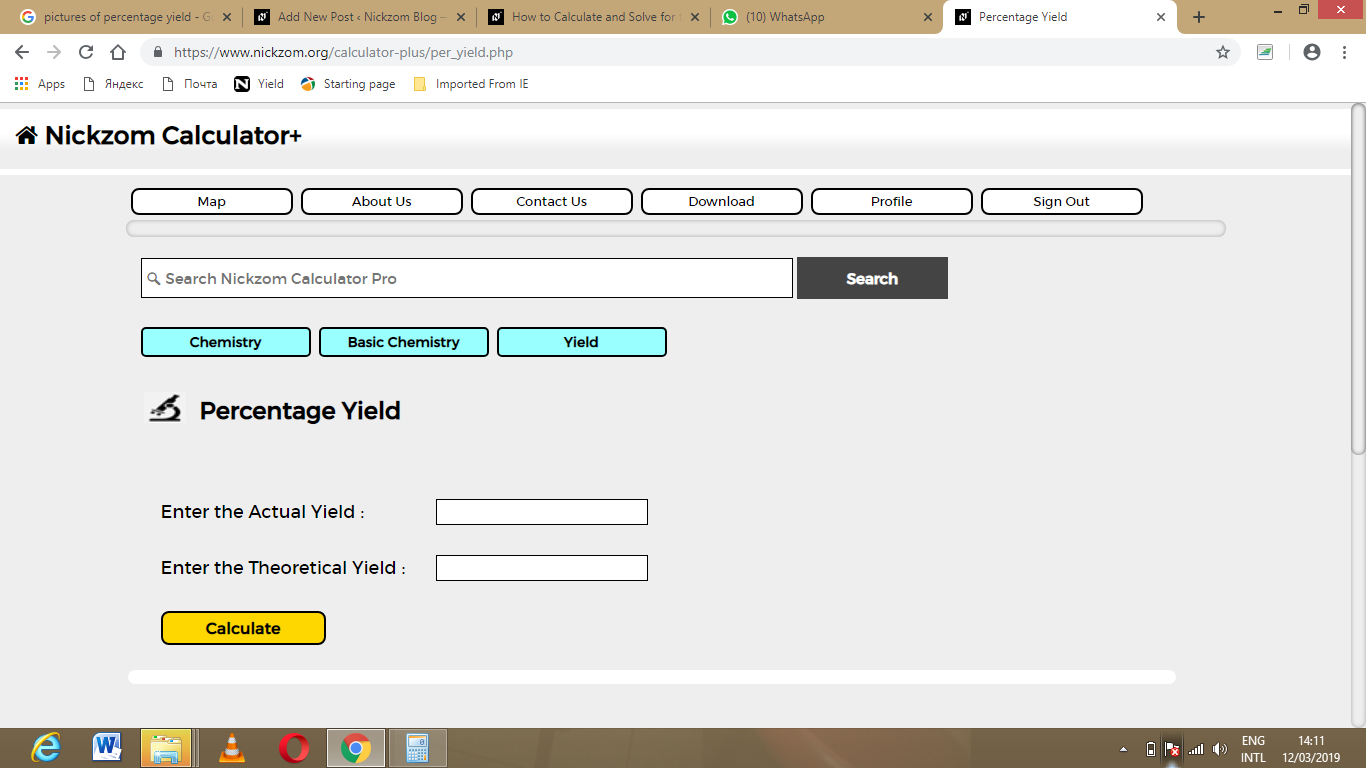Now, enter the values appropriately and accordingly for the parameters as required by the example above where the actual yield is 12 and theoretical yield is 26.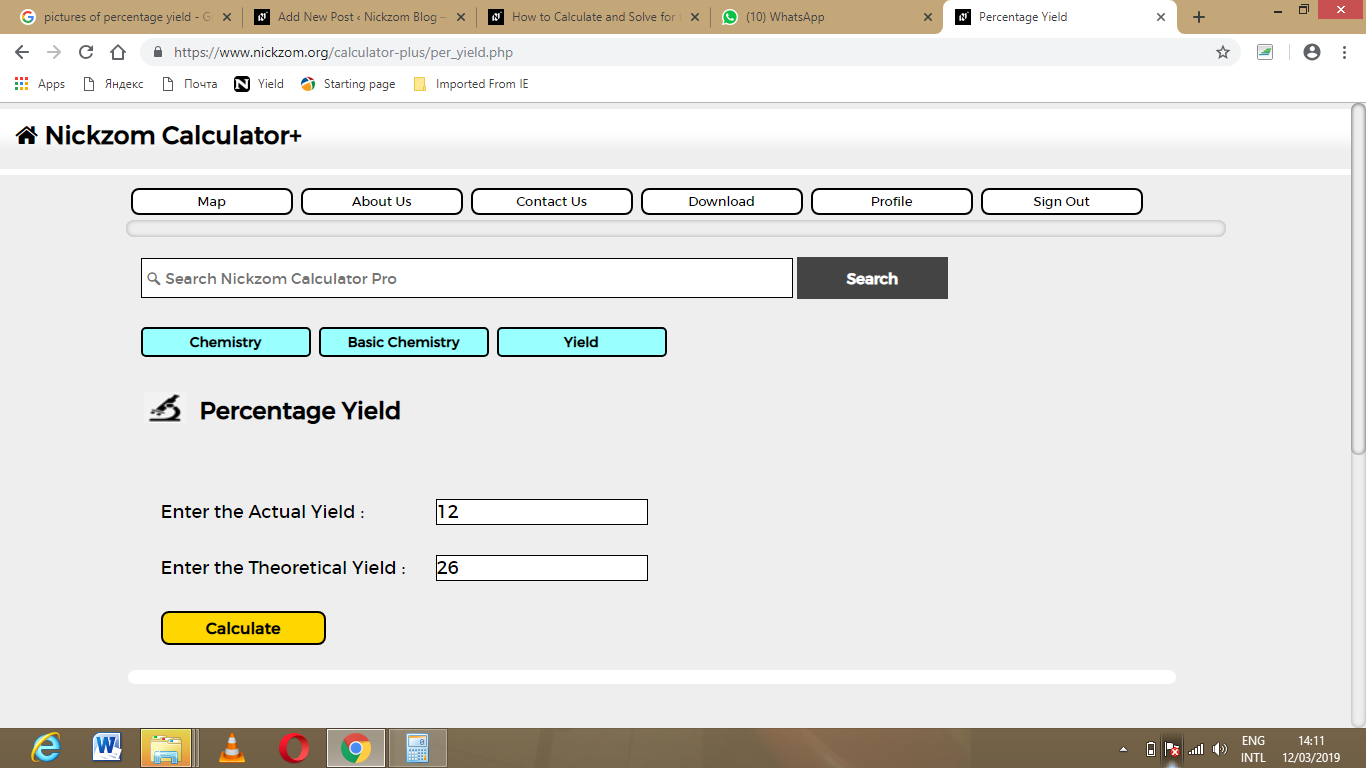Finally, click on Calculate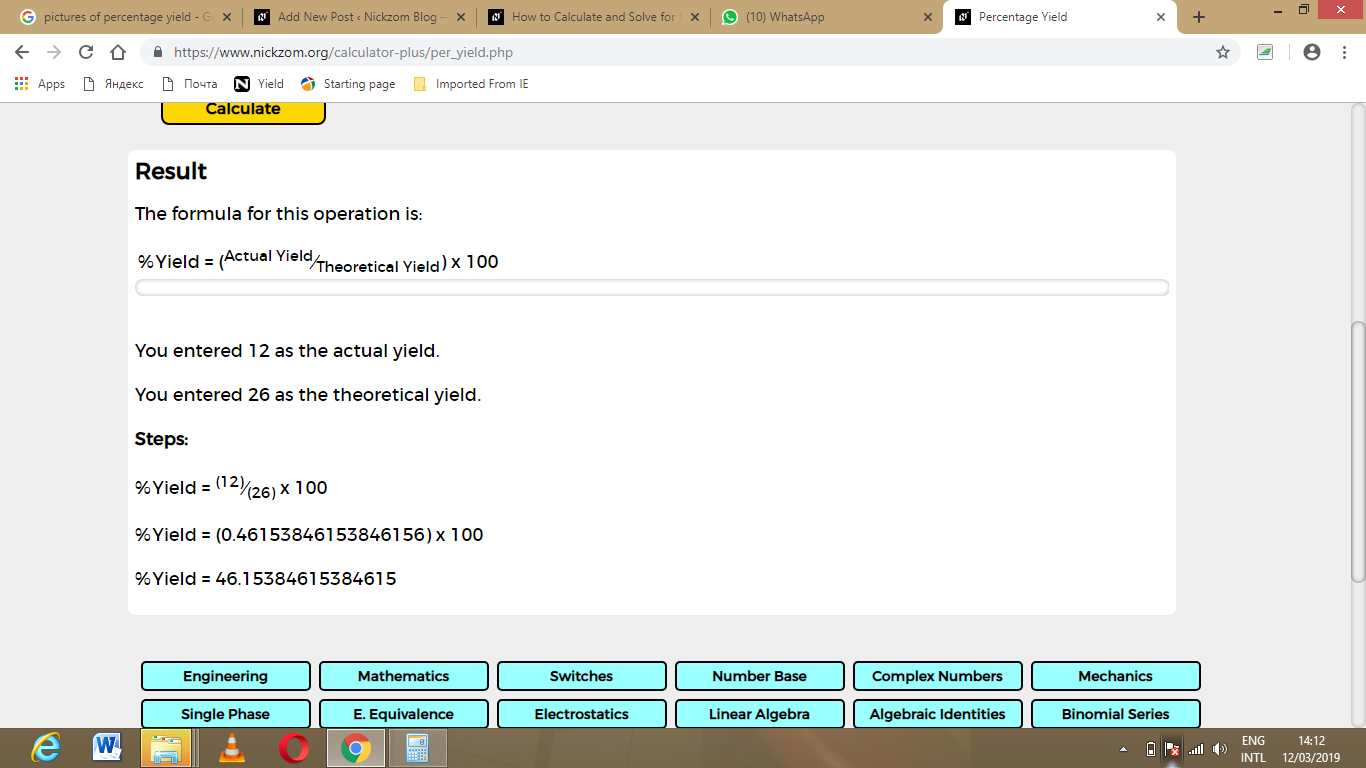As you can see from the screenshot above, Nickzom Calculator – The Calculator Encyclopedia solves for the percentage yield and presents the formula, workings and steps too.